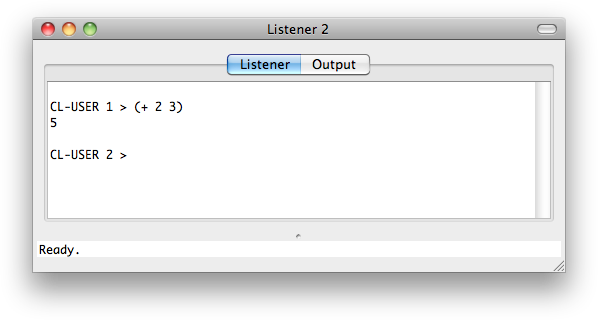## Getting Started

Unlike many other computer languages Lisp is interactive. It includes a window called the Listener which you can use like a calculator. You can type a Lisp expression at the ">" prompt, press return, and the system will evaluate it and show you the answer.

For example, to add two numbers together we can type:As you probably guessed (+ 2 3) is Lisp's way of adding the numbers 2 and 3, so it displays the result 5. The second prompt shows that it's ready for more.

In Lisp + is called a procedure. It is the operation to add numbers. In normal maths we write 2 + 3, but in Lisp we put the procedure name first, followed by the arguments, with the whole expression in brackets. This is called prefix notation, and although it seems strange, it's one of the best things about Lisp.

One advantage of prefix notation is that in normal maths notation, to add three numbers together we have to write:

`2 + 3 + 4`

but in Lisp we can write:

`CL-USER 3 > (+ 2 3 4)9`

### Arithmetic procedures - +, -, *, and /

Here are some other examples of simple Lisp procedures for working with numbers:

 Function Description Example + Add (+ 2 3 4) - Subtract (- 7 3) * Multiply (* 12 3) / Divide (/ 144 12)

Expressions can be nested; in other words, the arguments in an expression can themselves be expressions:

`CL-USER 4 > (/ (- 7 1) (- 4 2))3`

Another beauty of prefix notation is that it allows us to express almost everything we need, from simple calculations to complicated programs.

### Random numbers - random

The random procedure returns a random integer from 0 up to one less than its argument; for example:

```CL-USER > (random 10)
3

CL-USER > (random 10)
7```

Exercises

1. Write the Lisp expressions for the following maths expressions, and try evaluating them:

```2*3 + 7*83*4*5

-1 * 2 + 3```

2. Write an expression that will give a random dice throw from 1 to 6.

Previous: Debugging in LispWorks

Next: Lists